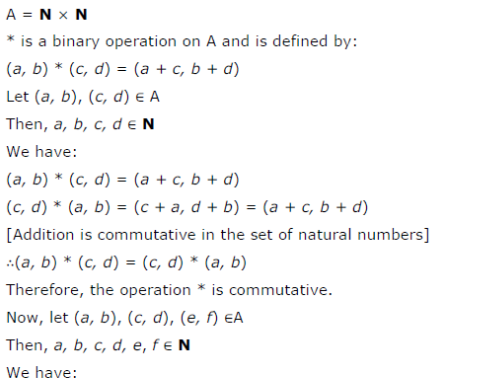# Let A = N x N and * be the binary operation on A defined by (a, b) * (c, d) = (a + c, b + d)

Let A = N x N and * be the binary operation on A defined by (a, b) * (c, d) = (a + c, b + d)
Show that * is commutative and associative. Find the identity element for * on A, if any.